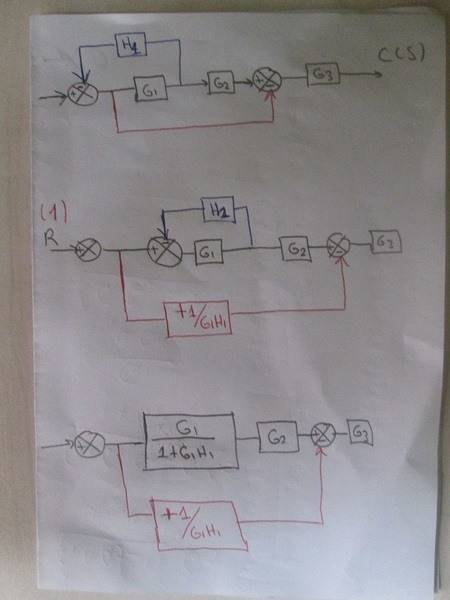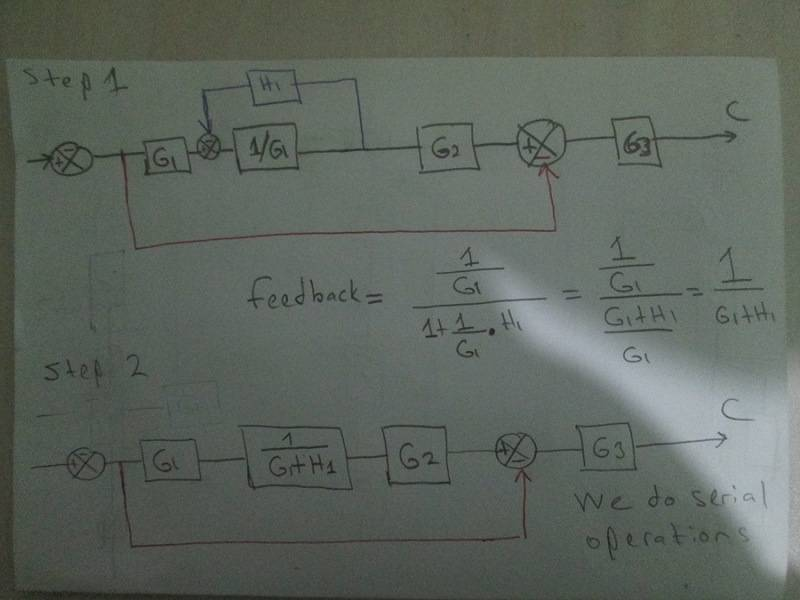# A block diagram reduction [sign error]

mech-eng
I try to solve a block diagram reduction example but when I did the operations, sign of one operation is incorrect. I tried to understand why it is so? Would somebody like to help me.

(1) First I carry the feedback into the parallel line.
(2) I add +1/G1H1 in the parallel line because I thought the orinal signal is that signal entering the parallel line.

3) I did serial and parallel operations but the result is incorrect by just a sign.

T(s)=G3 +G1G2G3/1+G1H1 which is the correct answer.

But the result I found by myself is
T(s)=-G3+G1G2G3/1+G1H1 which is the wrong answer by a sign.Thank you.

Phellippe Marques
I am really not aware of the movement you did from the first to the second pic, moving the feedback.

To solve this problem first I would move G1 to the right, we would have G1G2 and G1H1.
Then I would use the feedback formula to H1 and the unit.
Then I would make the parallel formula with the red wire and G1G2.

•mech-eng
mech-eng
I am really not aware of the movement you did from the first to the second pic, moving the feedback.

To solve this problem first I would move G1 to the right, we would have G1G2 and G1H1.
Then I would use the feedback formula to H1 and the unit.
Then I would make the parallel formula with the red wire and G1G2.

I have also solved this but moving feed back is an interesting way for me. But now I do not understand your method. Would you like to explain how you have G1H1 when you move G1 to the right and, of course out of the feed back.

Thank you.

mech-eng
It is confusing for me to mount redline under the upper feedback. If I attach redline under the upper feedback does the system change or the signal going through redline change?

Mentor
Are all those step relevant for the sign of G3, which seems independent of the previous operations? Where does the minus sign come from?

mech-eng
Are all those step relevant for the sign of G3, which seems independent of the previous operations? Where does the minus sign come from?

There is no problem with G3 or its sign. It is the last block and only serial to all the system before itself.

The problem is that how to put upper feed back inside the red line and while doing this how this line affects?

Thank you.

Mentor
There is no problem with G3 or its sign.
The first post suggests that the sign there is the problem.

I don't understand the problem.

Homework Helper
Gold Member
I assume that you left some parentheses off the denominator of your answer.

With that in mind, I got the same answer that you did. I think that the sign of the "correct" answer in the OP is wrong.

But I don't see how you got your answer. When I move the feed-forward take-off back in figure 2, I get 1/(1+G1H1) on the feed-forward signal.

You can move the take-off point of the feed-forward signal in front of the summation block or after the G1 block.

After the G1 block: The feed-forward signal gets a gain block 1/G1

In front of the summation block: The feed-forward signal gets a gain block 1/(1+G1H1)
You can see this by
1) first moving it after the G1 block as above, giving it a block 1/G1
2) consolidating the feedback to a single block K = G1/(1+G1H1);
3) move the take-off point in front of K, giving it a block K/G1 = 1/(1+G1H1)
I don't see how you got your feed-forward block 1/G1H1. I don't agree with that.

Last edited:
•mfb
mech-eng
The first post suggests that the sign there is the problem.

I don't understand the problem.

The problem is actually two part.

2. I tried to do it intuitively from the result, but parallel line enters the summing joint with negative sign. So the result of last step in the picture
[ G1G2/1+H1G1 - (+1/G1H1 ] * G3 there is substraction in the rectangular paranthesis because of negative summing of parallel line. I am asking about this.

Thank you.

mech-eng
In front of the summation block: The feed-forward signal gets a gain block 1/(1+G1H1)
You can see this by
1) first moving it after the G1 block as above, giving it a block 1/G1
2) consolidating the feedback to a single block K = G1/(1+G1H1);
3) move the take-off point in front of K, giving it a block K/G1 = 1/(1+G1H1)
I don't see how you got your feed-forward block 1/G1H1. I don't agree with that.

It seems this is what I want to success. But I cannot do the operations well. Something is wrong. Would you like to explain where I am wrong?Thank you.

Homework Helper
Gold Member
Step 1 is wrong. The signal going straight through now has a transform G1*1/G1 = I up to the feedback tap-point, which is wrong. It must still have the G1 transformation.

PS. People can discuss this more clearly if you label the signal points so we can say that a signal is being moved from point pm to point pn.

•mech-eng
mech-eng
Step 1 is wrong. The signal going straight through now has a transform G1*1/G1 = I, which is wrong. It must still have the G1 transformation.

PS. People can discuss this more clearly if you label the signal points so we can say that a signal is being moved from point pm to point pn.

This show even I do not know nature of signals and so I am making mistakes in calculating of feedbacks. How will I learn this?
Do we calculate feedbacks always with respect to forward signals? I first tried to calculate the feedback then I multiplied it with G1 which is wrong.

Thank you.

mech-eng
1) first moving it after the G1 block as above, giving it a block 1/G1.

Does not your step 1 corresponds to my step one in the last picture? If not, how can I do your step 1?

Thank you.